|

# 他在江南的山顶造了9间房只为看这一场雪!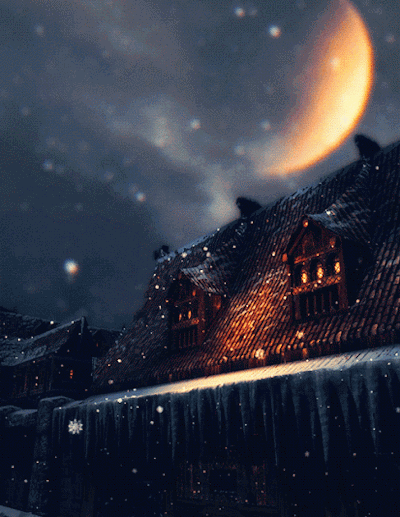富春江边的烟云缭绕，是相机拍不出的美

窗外，富春江内湖“芦茨”的夏天，一如既往的宁静。

一路开车进来，满目风雨。云，山水，竹林，光影将它们切碎了，打在蓝绿色的湖面上……

“我都想好了，上边要有9间房，才够朋友们一起住……来，尝尝着芦茨茶，不比碧螺春差。”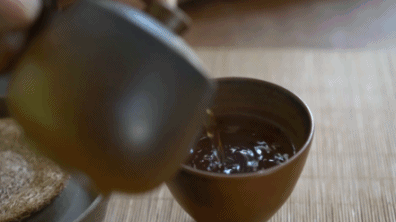“生活就跟这茶是一个道理，以前总以为好茶是要要到很远的地方去寻找的，大家都争着抢着的，稀有的，必定是好的。但其实，你想要的未必在远方，它可能就在你身边，比如回到乡村。”

练拳的人最讲究顺势而为，寻见芦茨村，做民宿“原乡芦茨”，也是因势而行。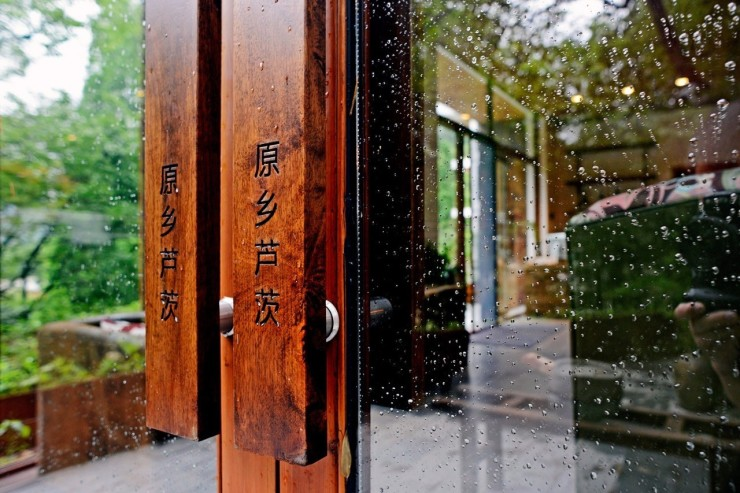人在芦茨，常常做这样的梦，梦里，我又回到了湖南老家。

奔跑在家乡的稻田上，有风，风里全是谷子的香气。狗叫声，鸡叫声……每每醒来，才会发觉，原来自己已身在别处。

在外面呆了那么多年，终还是习惯不了，在城市为了战胜不自卑耗尽身上的所有力气，每天背个笔记本，挣扎着要活出点什么，人模人样地扯着情怀和理想，其实呢，什么是生活早就忘了。

所以，遇见芦茨的时候，那个叫嚣着要去看看世界的我，又在再一次到了村里。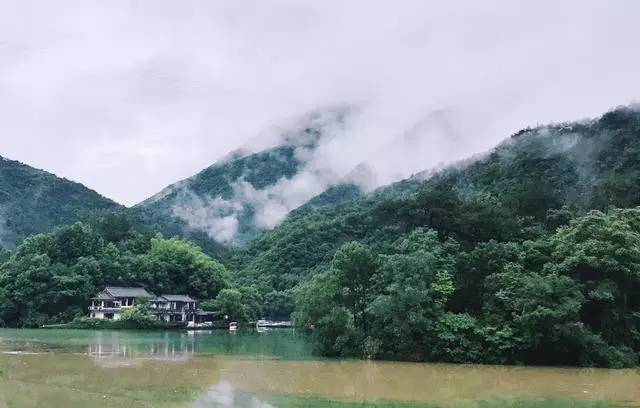租下了村民的二层农房做一屋民宿，“改造”却是差点意思，

于是请人整幢拆了，一切推倒重来。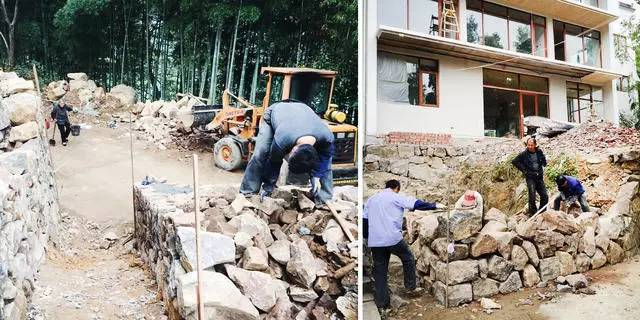三百万，六百平，

7间房，

却造了整整两年。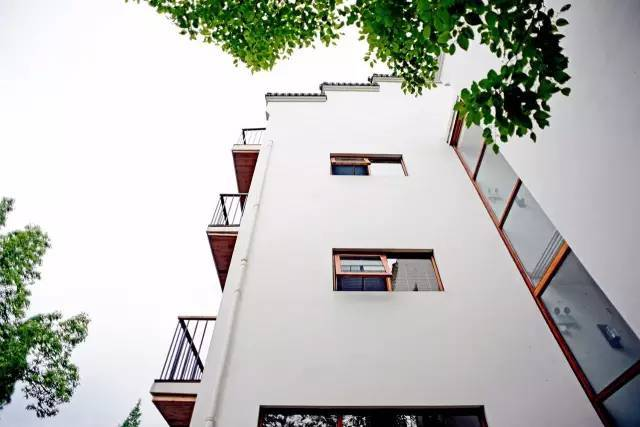两年里，换了三波设计师，有一半多的时间在重组装修团队，等合适的人来。

只是为了找到我想要的感觉。

只有这在地的师傅，才知道该怎么造当地的房子。

比如这墙壁的干砌法。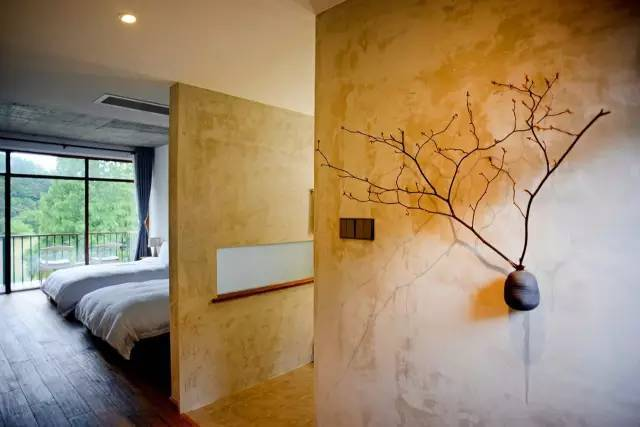最后和师傅抹了不知多少回，

才做出来的7间房，

每间望出去都是绿色，

镜子里也能看见树。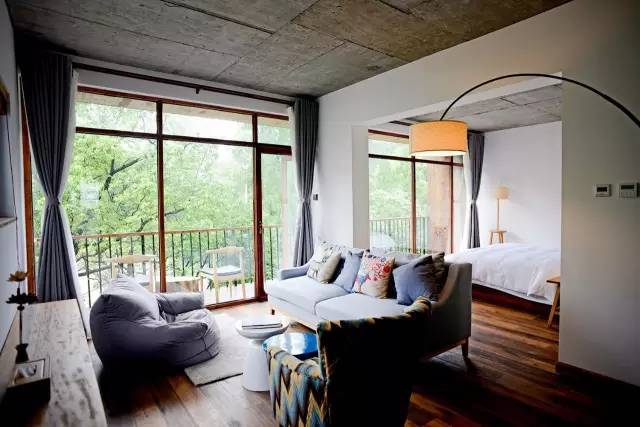窗外的树原本是不存在的。

装修时只砍掉了3根竹子，其他一律保留，施工方说这样没办法搭架子，

要砍树，我没有答应。

不是树妨碍我们了，是我们妨碍了树，树本来就在这，我们来打扰了它。

如今，它们都成了风景。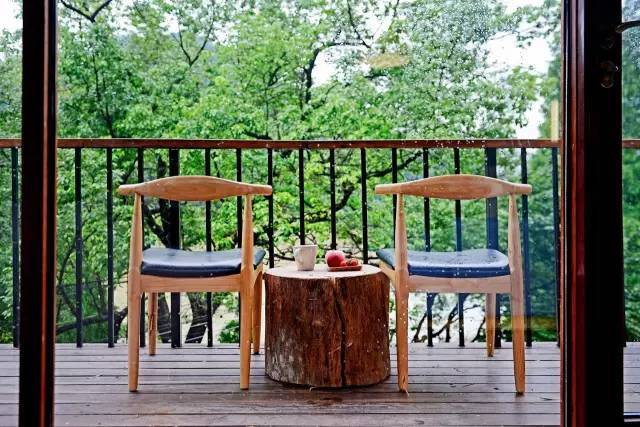不想模仿别人，就做自己喜欢的。

村民问为什么没有抽屉，为什么不吊顶，没办法回答，我却还是按自己想的做。

找来他们不要的篓和框，也找来一些有五六十年头的罐子作为花器，

砍一些树枝就可以做装饰。

家具是工厂定制的，实木，整块的木头。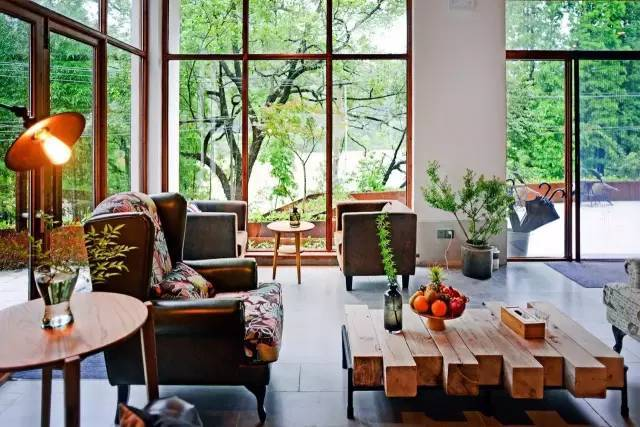拜托村长收集木头拼贴，粗糙的一面就留着，在弄一些铁架。

自己拼装拼装，涂料涂好，变成了茶几。

村长也赞叹不已，那些瓶罐，摆件，他们也觉得好看了，认可了。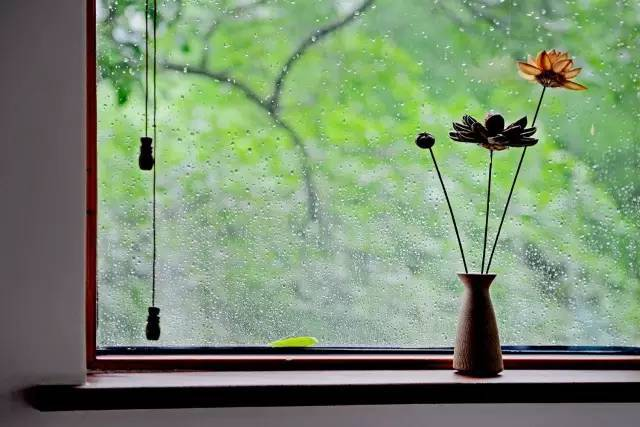做门的料，是从村里收来的旧木料。

本来已经全部做好了，是选择了现成的套门，但是又觉得不对，就拆了，

用旧物在现场重新制作。

感觉才到位。喜欢用村里的“野物”，这些不刻意的东西，自有它的趣味在，是人工出不来的。

比如，捡根木头就能做衣架。

搬个石头，就能做枯山水。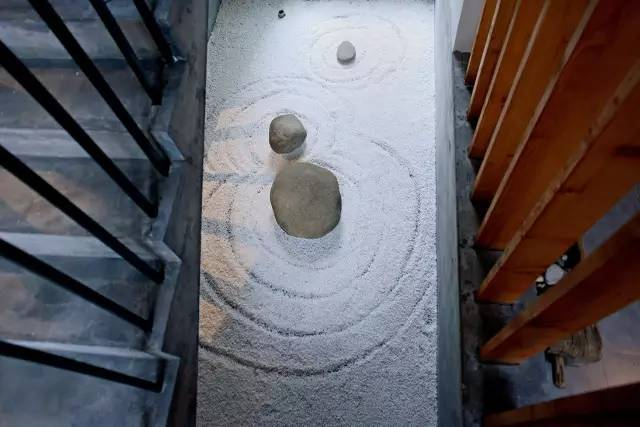可也并不只是执着这样的风格。

人住在里面，是不是舒服才是最重要的。

于是就有了这个，看似和周遭风马牛不相及的滑滑梯。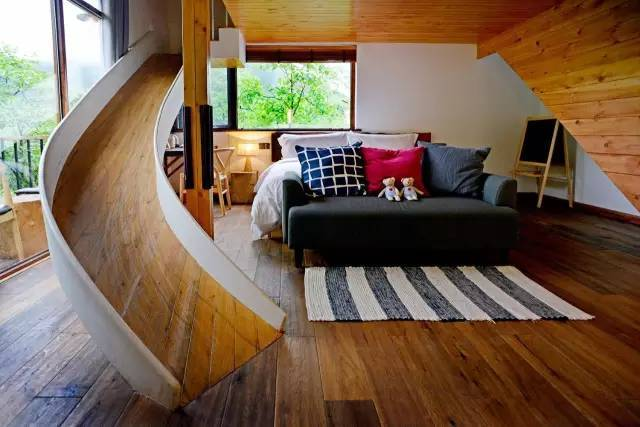有个孩子来了以后，在里面呆了一个下午也不出来。

当时想到做这个，是没有参考也没有标准的，设计师也没做过。

所以给出来的设计图也不对，要不就是角度不好，太陡危险，太缓又滑不起来。还比较容易挡住窗帘，影响走路。

最后自己上手画，折腾了5、6稿，居然成功了。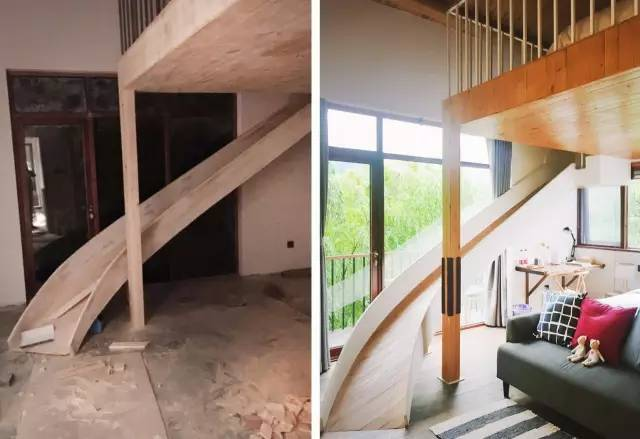滑滑梯对着的，

就是个大阳台，

下过雨的时候视野会很开阔。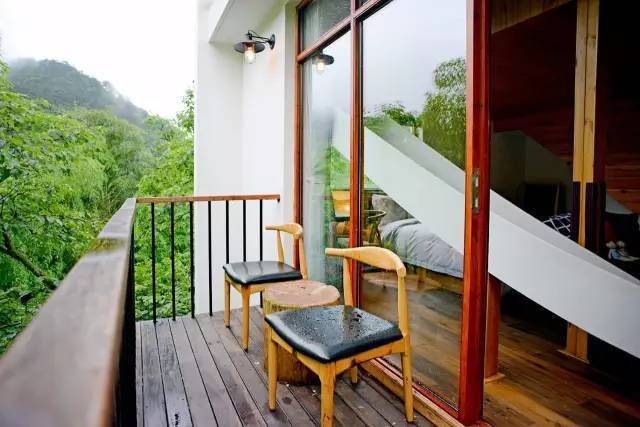房子是在地的师傅造的，里面的管家，也是村里人。

就是原先的房东一家：老傅夫妇和他们的儿子。

没有从外面请会泡咖啡的文青来当管家，还是那个原因，还有谁会比曾经住在这里的他们，更懂得这栋房子呢。甚至觉得他们比城里人更懂得生活，更懂得尊重自然时节。

什么季节做什么东西，像是很多糕点的做法，都是他们教我的。最喜欢下雨天，窝在书房泡茶看书。

如果恰好朋友来了，不能外出，那不如就来给大家下个厨吧。

记得小时候每逢下雨，大人就出不了工，全家人就会聚在一起，做桐也粑粑，烤火板栗……我喜欢这样温暖的感觉。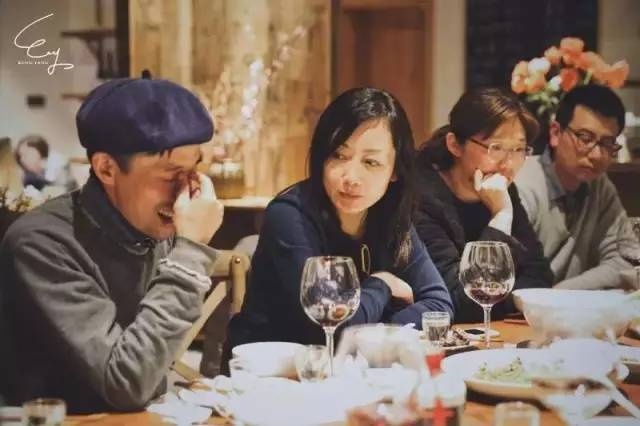如果天气足够晴朗，还是想带你去湖上划划船，顺便钓个鱼。

船是我从老渔民那里收来的闲置物，有50年的历史里，

你踩上去，就能在它上边看到使用过的痕迹。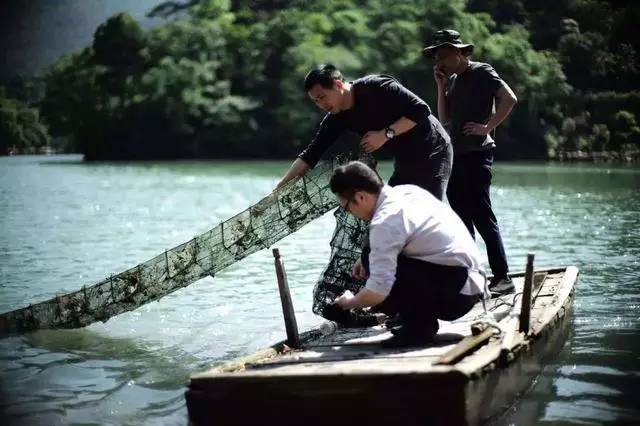但更多时候，

喜欢一个人飘在湖上，

什么也不做，

就看看天，看看山。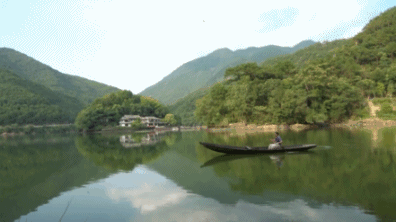总觉得山里的四季生活，我们还能再过得不一样一点。

所以去年冬天，在山顶偶遇初雪之后，便有了现在的芦茨白云源——一栋为了看雪而建的民宿。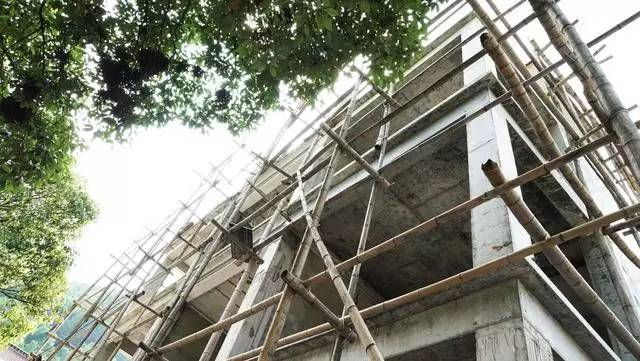建造中的原乡·白云源，在海拔600米的山顶。

站在富春江边的山顶。看鹅毛大雪飘落下来，一点点把周遭的草木给埋了起来，软绵绵，湿哒哒……坐在院子里，院子里放个炭火炉子，炉子上再放上几片年糕，温一壶米酒……

在山脚下的原乡芦茨所不能做到的，在山顶，可以。

雪在600米的海拔上，可以积的很厚，即使是身在江南，一年足可以看上个四五次。白云源设计概念图

会在房子边上做一个石头砌的无边泳池，把山泉水直接接到泳池里，夏日里淌一身清凉。还会用砖砌个面包炉子，和大家一起揉面，做窑烤面包。建筑主体旁边还有一座独立的小屋。我会把它改造成一个竹器和陶器的手工作坊。

当然最美妙的，还是看雪。饮茶煮雪

喜欢这样的四季分明，悠哉悠哉的日子。

其实很多年前，离开大山的时候，不曾想过还会回归农村。只是走着走着就到了这里。

有的朋友看淡红尘，对事实梳理，转而喜欢山里的淳朴，于我，并没有太大的转变，也没有蓦然回收的彻悟，只身在芦茨，看四时花木，听山涧虫鸣，这都是根植在我天性中的。

回过头看看已经走过的路，原来我想要的，不过是一阵闲适的清风。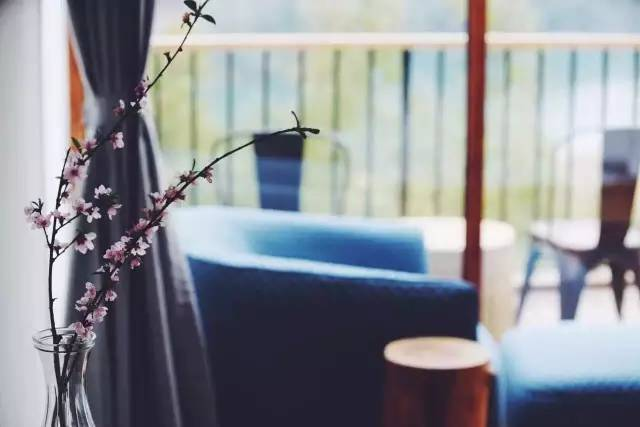不要让太多物质，

遮蔽住了我们本来清澈的脸庞。

你追寻的生活，

也许，

它就在你蓦然回首的地方。

`声明：本文由入驻焦点开放平台的作者撰写，除焦点官方账号外，观点仅代表作者本人，不代表焦点立场错误信息举报电话： 400-099-0099，邮箱：jubao@vip.sohu.com，或点此进行意见反馈，或点此进行举报投诉。`A B C D E F G H J K L M N P Q R S T W X Y Z
A - B - C - D - E
• A
• 鞍山
• 安庆
• 安阳
• 安顺
• 安康
• 澳门
• B
• 北京
• 保定
• 包头
• 巴彦淖尔
• 本溪
• 蚌埠
• 亳州
• 滨州
• 北海
• 百色
• 巴中
• 毕节
• 保山
• 宝鸡
• 白银
• 巴州
• C
• 承德
• 沧州
• 长治
• 赤峰
• 朝阳
• 长春
• 常州
• 滁州
• 池州
• 长沙
• 常德
• 郴州
• 潮州
• 崇左
• 重庆
• 成都
• 楚雄
• 昌都
• 慈溪
• 常熟
• D
• 大同
• 大连
• 丹东
• 大庆
• 东营
• 德州
• 东莞
• 德阳
• 达州
• 大理
• 德宏
• 定西
• 儋州
• 东平
• E
• 鄂尔多斯
• 鄂州
• 恩施
F - G - H - I - J
• F
• 抚顺
• 阜新
• 阜阳
• 福州
• 抚州
• 佛山
• 防城港
• G
• 赣州
• 广州
• 桂林
• 贵港
• 广元
• 广安
• 贵阳
• 固原
• H
• 邯郸
• 衡水
• 呼和浩特
• 呼伦贝尔
• 葫芦岛
• 哈尔滨
• 黑河
• 淮安
• 杭州
• 湖州
• 合肥
• 淮南
• 淮北
• 黄山
• 菏泽
• 鹤壁
• 黄石
• 黄冈
• 衡阳
• 怀化
• 惠州
• 河源
• 贺州
• 河池
• 海口
• 红河
• 汉中
• 海东
• 怀来
• I
• J
• 晋中
• 锦州
• 吉林
• 鸡西
• 佳木斯
• 嘉兴
• 金华
• 景德镇
• 九江
• 吉安
• 济南
• 济宁
• 焦作
• 荆门
• 荆州
• 江门
• 揭阳
• 金昌
• 酒泉
• 嘉峪关
K - L - M - N - P
• K
• 开封
• 昆明
• 昆山
• L
• 廊坊
• 临汾
• 辽阳
• 连云港
• 丽水
• 六安
• 龙岩
• 莱芜
• 临沂
• 聊城
• 洛阳
• 漯河
• 娄底
• 柳州
• 来宾
• 泸州
• 乐山
• 六盘水
• 丽江
• 临沧
• 拉萨
• 林芝
• 兰州
• 陇南
• M
• 牡丹江
• 马鞍山
• 茂名
• 梅州
• 绵阳
• 眉山
• N
• 南京
• 南通
• 宁波
• 南平
• 宁德
• 南昌
• 南阳
• 南宁
• 内江
• 南充
• P
• 盘锦
• 莆田
• 平顶山
• 濮阳
• 攀枝花
• 普洱
• 平凉
Q - R - S - T - W
• Q
• 秦皇岛
• 齐齐哈尔
• 衢州
• 泉州
• 青岛
• 清远
• 钦州
• 黔南
• 曲靖
• 庆阳
• R
• 日照
• 日喀则
• S
• 石家庄
• 沈阳
• 双鸭山
• 绥化
• 上海
• 苏州
• 宿迁
• 绍兴
• 宿州
• 三明
• 上饶
• 三门峡
• 商丘
• 十堰
• 随州
• 邵阳
• 韶关
• 深圳
• 汕头
• 汕尾
• 三亚
• 三沙
• 遂宁
• 山南
• 商洛
• 石嘴山
• T
• 天津
• 唐山
• 太原
• 通辽
• 铁岭
• 泰州
• 台州
• 铜陵
• 泰安
• 铜仁
• 铜川
• 天水
• 天门
• W
• 乌海
• 乌兰察布
• 无锡
• 温州
• 芜湖
• 潍坊
• 威海
• 武汉
• 梧州
• 渭南
• 武威
• 吴忠
• 乌鲁木齐
X - Y - Z
• X
• 邢台
• 徐州
• 宣城
• 厦门
• 新乡
• 许昌
• 信阳
• 襄阳
• 孝感
• 咸宁
• 湘潭
• 湘西
• 西双版纳
• 西安
• 咸阳
• 西宁
• 仙桃
• 西昌
• Y
• 运城
• 营口
• 盐城
• 扬州
• 鹰潭
• 宜春
• 烟台
• 宜昌
• 岳阳
• 益阳
• 永州
• 阳江
• 云浮
• 玉林
• 宜宾
• 雅安
• 玉溪
• 延安
• 榆林
• 银川
• Z
• 张家口
• 镇江
• 舟山
• 漳州
• 淄博
• 枣庄
• 郑州
• 周口
• 驻马店
• 株洲
• 张家界
• 珠海
• 湛江
• 肇庆
• 中山
• 自贡
• 资阳
• 遵义
• 昭通
• 张掖
• 中卫

1室1厅1厨1卫1阳台

1
2
3
4
5

0
1
2

1

1

0
1
2
3报名成功，资料已提交审核A B C D E F G H J K L M N P Q R S T W X Y Z
A - B - C - D - E
• A
• 鞍山
• 安庆
• 安阳
• 安顺
• 安康
• 澳门
• B
• 北京
• 保定
• 包头
• 巴彦淖尔
• 本溪
• 蚌埠
• 亳州
• 滨州
• 北海
• 百色
• 巴中
• 毕节
• 保山
• 宝鸡
• 白银
• 巴州
• C
• 承德
• 沧州
• 长治
• 赤峰
• 朝阳
• 长春
• 常州
• 滁州
• 池州
• 长沙
• 常德
• 郴州
• 潮州
• 崇左
• 重庆
• 成都
• 楚雄
• 昌都
• 慈溪
• 常熟
• D
• 大同
• 大连
• 丹东
• 大庆
• 东营
• 德州
• 东莞
• 德阳
• 达州
• 大理
• 德宏
• 定西
• 儋州
• 东平
• E
• 鄂尔多斯
• 鄂州
• 恩施
F - G - H - I - J
• F
• 抚顺
• 阜新
• 阜阳
• 福州
• 抚州
• 佛山
• 防城港
• G
• 赣州
• 广州
• 桂林
• 贵港
• 广元
• 广安
• 贵阳
• 固原
• H
• 邯郸
• 衡水
• 呼和浩特
• 呼伦贝尔
• 葫芦岛
• 哈尔滨
• 黑河
• 淮安
• 杭州
• 湖州
• 合肥
• 淮南
• 淮北
• 黄山
• 菏泽
• 鹤壁
• 黄石
• 黄冈
• 衡阳
• 怀化
• 惠州
• 河源
• 贺州
• 河池
• 海口
• 红河
• 汉中
• 海东
• 怀来
• I
• J
• 晋中
• 锦州
• 吉林
• 鸡西
• 佳木斯
• 嘉兴
• 金华
• 景德镇
• 九江
• 吉安
• 济南
• 济宁
• 焦作
• 荆门
• 荆州
• 江门
• 揭阳
• 金昌
• 酒泉
• 嘉峪关
K - L - M - N - P
• K
• 开封
• 昆明
• 昆山
• L
• 廊坊
• 临汾
• 辽阳
• 连云港
• 丽水
• 六安
• 龙岩
• 莱芜
• 临沂
• 聊城
• 洛阳
• 漯河
• 娄底
• 柳州
• 来宾
• 泸州
• 乐山
• 六盘水
• 丽江
• 临沧
• 拉萨
• 林芝
• 兰州
• 陇南
• M
• 牡丹江
• 马鞍山
• 茂名
• 梅州
• 绵阳
• 眉山
• N
• 南京
• 南通
• 宁波
• 南平
• 宁德
• 南昌
• 南阳
• 南宁
• 内江
• 南充
• P
• 盘锦
• 莆田
• 平顶山
• 濮阳
• 攀枝花
• 普洱
• 平凉
Q - R - S - T - W
• Q
• 秦皇岛
• 齐齐哈尔
• 衢州
• 泉州
• 青岛
• 清远
• 钦州
• 黔南
• 曲靖
• 庆阳
• R
• 日照
• 日喀则
• S
• 石家庄
• 沈阳
• 双鸭山
• 绥化
• 上海
• 苏州
• 宿迁
• 绍兴
• 宿州
• 三明
• 上饶
• 三门峡
• 商丘
• 十堰
• 随州
• 邵阳
• 韶关
• 深圳
• 汕头
• 汕尾
• 三亚
• 三沙
• 遂宁
• 山南
• 商洛
• 石嘴山
• T
• 天津
• 唐山
• 太原
• 通辽
• 铁岭
• 泰州
• 台州
• 铜陵
• 泰安
• 铜仁
• 铜川
• 天水
• 天门
• W
• 乌海
• 乌兰察布
• 无锡
• 温州
• 芜湖
• 潍坊
• 威海
• 武汉
• 梧州
• 渭南
• 武威
• 吴忠
• 乌鲁木齐
X - Y - Z
• X
• 邢台
• 徐州
• 宣城
• 厦门
• 新乡
• 许昌
• 信阳
• 襄阳
• 孝感
• 咸宁
• 湘潭
• 湘西
• 西双版纳
• 西安
• 咸阳
• 西宁
• 仙桃
• 西昌
• Y
• 运城
• 营口
• 盐城
• 扬州
• 鹰潭
• 宜春
• 烟台
• 宜昌
• 岳阳
• 益阳
• 永州
• 阳江
• 云浮
• 玉林
• 宜宾
• 雅安
• 玉溪
• 延安
• 榆林
• 银川
• Z
• 张家口
• 镇江
• 舟山
• 漳州
• 淄博
• 枣庄
• 郑州
• 周口
• 驻马店
• 株洲
• 张家界
• 珠海
• 湛江
• 肇庆
• 中山
• 自贡
• 资阳
• 遵义
• 昭通
• 张掖
• 中卫• 手机• 分享
• 设计
免费设计
• 计算器
装修计算器
• 入驻
合作入驻
• 联系
联系我们
• 置顶
返回顶部